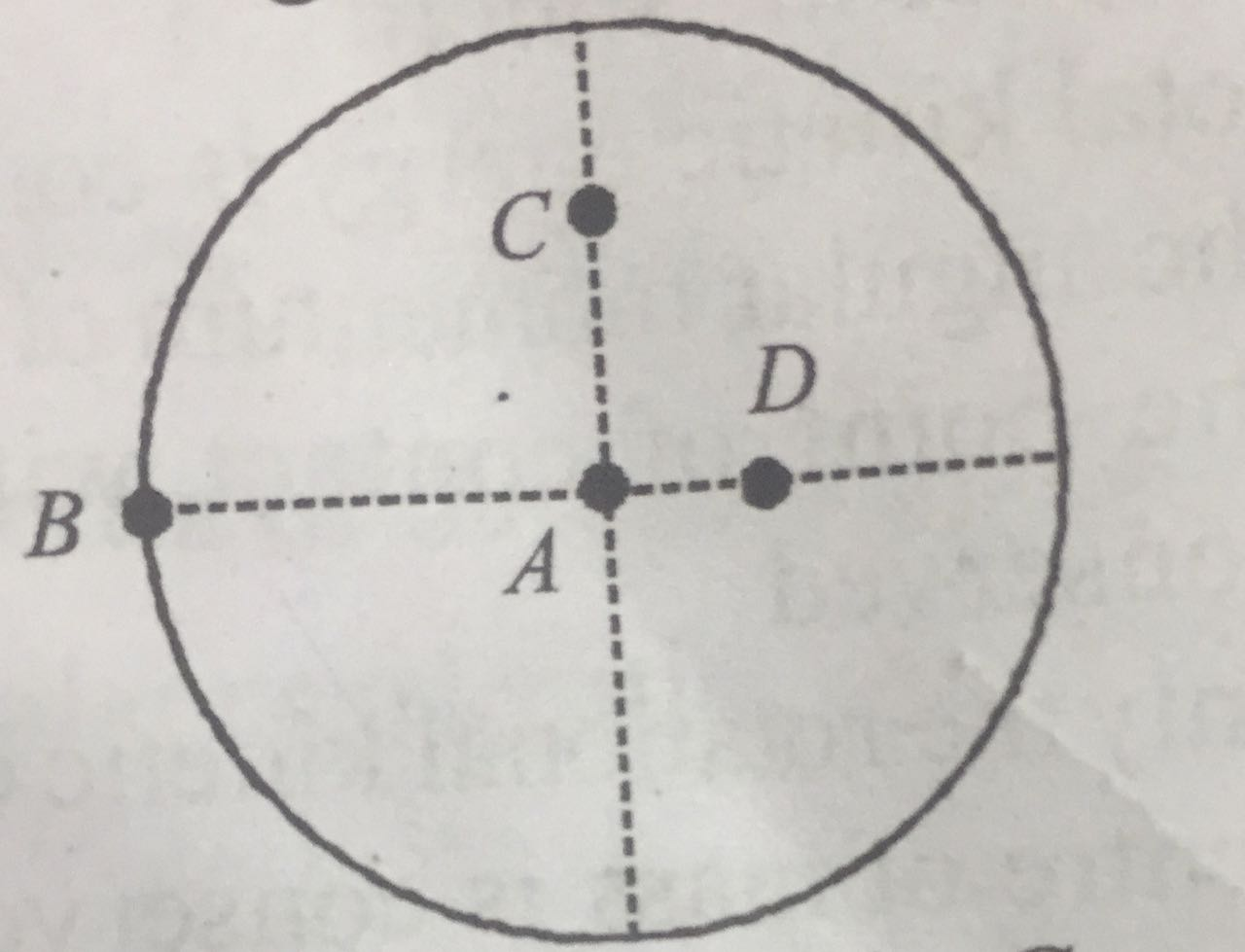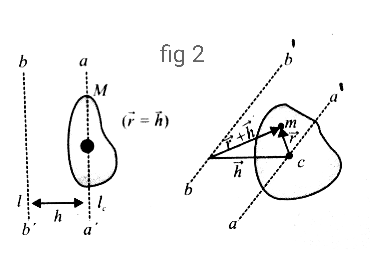# The moment of inertia of a uniform circular disc is maximum about an axis perpendicular to the disc and passing throughOption 1) B Option 2) C Option 3) D Option 4) A

V varun

b

P Plabita

as discussed in

Paraller Axis Theorem -- wherein

is axis parallel to & an axis passing through centre of mass.

According to parallel axis theorem,

R is maximum for point B, so Imax is about B.

Option 1)

B

This option is correct.

Option 2)

C

This option is incorrect.

Option 3)

D

This option is incorrect.

Option 4)

A

This option is incorrect.

Exams
Articles
Questions